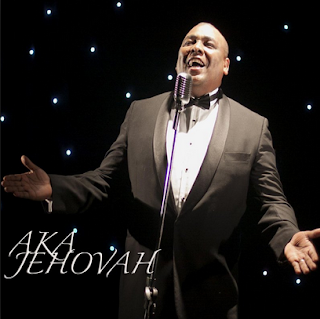# Solfa notation of Aka Akaya(Soprano, Alto and Tenor part)

###This is the tonic solf in parts(treble, Alto and tenor) of Aka Akaya, a popular worship song in Nigeria.

A lot of us want to learn to sing our parts perfectly. Whether you're a soprano, alto or tenor singer; you can only get better at it as you begin to practice more on a daily basis.

### Soprano - Aka Akaya

s s m f s
m m t d r t d l
d d t d r
m r d d r d l s
m m r d s
d t s t m s f
l l m f s
d d s s l s f m

Tenor - Aka Akaya

d d s l d
s s m m f m r d
m m r m f
s f m m f m r d
s s f m d
m r d r t d l
d d s l t
m m t d r d l s

m m d r m
d d l l t l s f
l l s l t
d t l l t s f m
d d t l m
l s m s m f r
f f d r m
l l m f s f r d